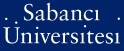# Bilinearity rank of the cone of positive polynomials and related cones

Rudolf, Gabor and Noyan, Nilay and Papp, David and Alizadeh, Farid (2010) Bilinearity rank of the cone of positive polynomials and related cones. (Accepted/In Press)There is a more recent version of this item available.Preview
PDF (This is a RoMEO green publisher -- author can archive pre-print (ie pre-refereeing) and post-print (ie final draft post-refereeing)) - Requires a PDF viewer such as GSview, Xpdf or Adobe Acrobat Reader
435Kb

## Abstract

For a proper cone K ⊂ Rn and its dual cone K the complementary slackness condition xT s = 0 defines an n-dimensional manifold C(K) in the space { (x, s) | x ∈ K, s ∈ K^* }. When K is a symmetric cone, this manifold can be described by a set of n bilinear equalities. When K is a symmetric cone, this fact translates to a set of n linearly independent bilinear identities (optimality conditions) satisfied by every (x, s) ∈ C(K). This proves to be very useful when optimizing over such cones, therefore it is natural to look for similar optimality conditions for non-symmetric cones. In this paper we define the bilinearity rank of a cone, which is the number of linearly independent bilinear identities valid for the cone, and describe a linear algebraic technique to bound this quantity. We examine several well-known cones, in particular the cone of positive polynomials P2n+1 and its dual, the closure of the moment cone M2n+1, and compute their bilinearity ranks. We show that there are exactly four linearly independent bilinear identities which hold for all (x,s) ∈ C(P2n+1), regardless of the dimension of the cones. For nonnegative polynomials over an interval or half-line there are only two linearly independent bilinear identities. These results are extended to trigonometric and exponential polynomials.

Item Type: Article Optimality conditions, positive polynomials, complementarity slackness, bilinearity rank, bilinear cones Q Science > Q Science (General) 14714 Nilay Noyan 13 Oct 2010 10:17 25 Jul 2019 16:31

Repository Staff Only: item control page